# 【A-level化学】有机化学中涉及的化学反应大总结

2020-06-02  正领国际教育
1.Alkane-chloroalkane reagents: Cl2conditions: UV lightmechanism: free radical substitutionequation: RH + Cl2=RCl + HCl 2.Alkene- polyalkene Conditions: low T,

1.Alkane-chloroalkane

reagents: Cl2

conditions: UV light

equation: RH + Cl2=RCl + HCl

2.Alkene- polyalkene

Conditions: low T, high p.

Equation: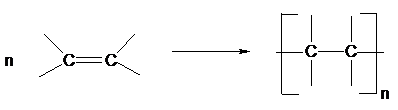3.Alkene-bromoalkane

Reagent: HX(g)

Conditions: room T

Equation: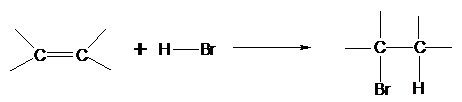4.Alkene-dibromoalkane

Reagent: Br2 in water or in an organic solvent

Conditions: room T

Equation: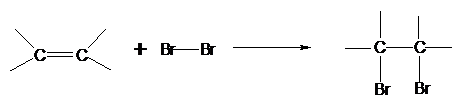5.Alkene-alkylhydrogensulphate

Reagent: concentrated sulphuric acid

Conditions: cold

Equation: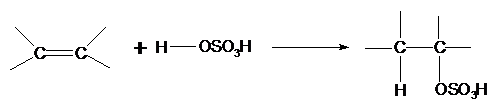6.Alkylhydrogensulphate-alcohol

Reagent: water

Conditions: warm

Equation: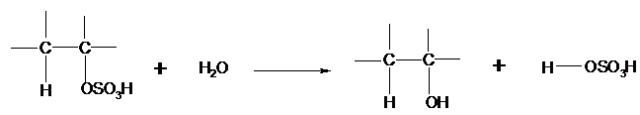Type of reaction: hydrolysis

7.Alkene-alcohol

Reagent: steam

Conditions: 300oC, 60 atm, H3PO4 catalyst

Equation: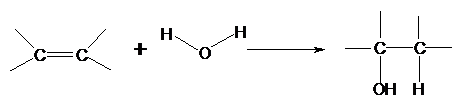Type of reaction: hydration

8.Haloalkane-alcohol

Reagent: NaOH(aq) or KOH(aq)

Conditions: warm under reflux

Equation: R-X + OH-= R-OH + X-

Type of reaction: nucleophilic substitution

9.Haloalkane-nitrile

Reagent: KCN in aqueous ethanol

Conditions: boil under reflux

Equation: R-X + CN- =R-CN + X-

Type of reaction: nucleophilic substitution

10.Haloalkane-Amine

Reagent: ammonia in ethanol in a sealed tube

Conditions: heat

Equation: R-X + 2NH3=R-NH2 + NH4X

Type of reaction: nucleophilic substitution

11.Haloalkane-alkene

Reagent: KOH in ethanol

Conditions: heat

Equation: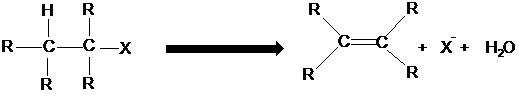Type of reaction: elimination

12.Primary alcohol-aldehyde

Reagent: potassium dichromate and dilute sulphuric acid

Conditions: warm, distillation

Equation: RCH2OH + [O]=RCHO + H2O

Type of reaction: mild oxidation

13.Secondary alcohol-ketone

Reagent: potassium dichromate and dilute sulphuric acid

Conditions: heat, distillation

Equation: R1CH(OH)R2 + [O]=R1COR2 + H2O

Type of reaction: oxidation

14.aldehyde-carboxylic acid

Reagent: potassium dichromate and dilute sulphuric acid

Conditions: heat, reflux

Equation: R-CHO + [O]=R-COOH

Type of reaction: oxidation

15.Alcohols-alkenes

Reagent: concentrated sulphuric acid

Conditions: heat

Equation: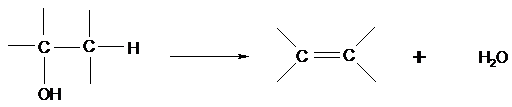Type of reaction: elimination

16.glucose-ethanol

reagent: yeast

conditions: 35 – 55℃, no air

equation: C6H12O6=2C2H5OH + 2CO2

type of reaction: fermentation